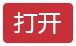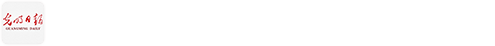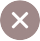﻿ Torus _推荐 _光明网
• 您想去哪里？
﻿

# Torus

2019-01-17 12:08　来源：留学杂志　陈一帆
2019-01-17 12:08:25来源：留学杂志作者：陈一帆责任编辑：王宏泽

1.0 Introduction

1.1Definition

In geometry, a torus is generated by revolving a circle in three-dimensional space about an axis coplanar with the circle. If the axis of revolution does not cover the circle, the surface has a ring shape and is called a torus of revolution.

Topologically, a torus is a closed surface defined as the Cartesian product of two circles: S×S . This topological torus is also often called the Clifford torus.

(The picture of a torus is shown above.)

2.0 Ways to get a torus

2.1 Geometry

A torus is defined in the coordinate system by:

x(θ,φ)=(R+r.cosθ ) cosφ

y(θ,φ)=(R+r.cosθ ) cosφ

z(θ,φ)=r.sinθ

where θ,φ are the values of angles which make a full circle, and R is the distance between the center of the tube and the center of the tube.

Let r equals to the radius of the inner loop

When R>r, it is called ring torus or anchor ring.

When R=r, it is called horn torus.

When R

2.2Topology

The torus can also be described as a quotient of the Cartesian plane under the identifications: (0,y)~(1,y),(x,0)~(x,1), or as the quotient of the unit square by pasting the opposite edges together, described as a fundamental polygon ABA_(-1) B_(-1).

3.0Torus

3.1 Property

The methods used to construct a torus in MALAB is triangulation. Usually, in Topology, we use triangle to form an object that is homeomorphic to the object that we expect to have. (Homeomorphism is a special property in Topology means that we can get an object through continuous map from the initial object.) 9 vertexes ad 18 triangles are used in total to form a torus. The total simplices is 54.

（A simplicial complex is a ﬁnite collection of simplices K such that

1.Every face of a simplex in K also belongs to K.

2.For any two simplices σ1 and σ2 in K, if σ1∩σ2≠φ, then σ1∩σ2 is a common face of both σ1 and σ2.）

The Betti number: betti0=1 ,betti1=2, betti2=1 (See in appendix A)

In addition, to divide a torus into regions, then it is always possible to color the regions with at least seven colors so that neighboring regions have different colors. (Compare to the four color theorem for a single plane)

3.2 N-dimensional torus

The torus has a generalization to higher dimensions, the n-dimensional torus, often called the n-torus or hypertorus for short. (This is one of two different meanings of the term "n-torus".) Recalling that the torus is the product space of two circles, the n-dimensional torus is the product of n circles. That is:

T^n=S^1×……×S^n

3.3 Application

Torus is widely used in famous games for building the maps which can make the map cyclic because torus has only one face in three-dimensional space. As in the classic computer game Asteroids (see in Appendix B), when you travel up through the top of the rectangle, you reappear at the bottom, and traveling through the right side causes you to appear on the left. This drawing, though not quite as appetizing as the donut, still shows us all the important topological properties of the torus.

4.0 Conclusion

Torus is a topological feature that ca be obtained in many ways including geometry and topology. In geometry, we mainly discuss how to get the volume using calculus, However, in topology, we care about the homology group and its homeomorphic class. People once said that a mathematician cannot tell the difference between the coffee cup and donut, because we can use the shape of coffee cup to make a donut by continuous map. Also, by using the properties of torus, we can make interesting small games like Asteroids.

陈一帆

年龄：15

城市：北京

就读学校：北师大二附中国际部

年级：高二

目标专业：应用数学

应用拓扑学对我来说是一个极大的挑战，过去所学的大多都是高等代数知识，像拓扑学这种将代数和图像结合起来的学科是我第一次接触。在学习拓扑学的同时我也在教授的指导下学习了线性代数，这次经历让我了解了什么叫真正的数学，也让我更加确定了未来学习应用数学的决心。本论文的主题为torus，这是一个拓扑学中重要的结构之一，此次自主研究也让我了解了拓扑结构在现实生活中的应用，第一次真正的将理论数学和应用数学结合起来。[责任编辑:王宏泽][值班总编推荐]

[值班总编推荐]

[值班总编推荐]